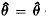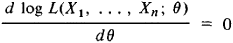# Maximum Likelihood Method

Also found in: Dictionary, Acronyms.

## maximum likelihood method

[′mak·sə·məm ′līk·lē‚hu̇d ‚meth·əd]
(statistics)
A technique in statistics where the likelihood distribution is so maximized as to produce an estimate to the random variables involved.

## Maximum Likelihood Method

a method of finding statistical estimates of the unknown parameters of a distribution. According to the maximum likelihood method, we select as the estimates of the parameters those values for which the data resulting from observations are “most likely.” It is assumed that the results of observations X1, …, Xn are mutually independent random variables with identical probability distributions, all depending on the same unknown parameter θ ε ө, where ө is the set of admissible values of θ. To assign an exact meaning to the concept of “most likely,” we proceed by introducing a function

L(x1, …, xn; θ) = p(x1; θ) … p(xn; θ)

where p(t;θ) for a continuous distribution is interpreted as the probability density of the random variable X, and in the discrete case as the probability that the random variable X takes the value t. The function L(X1, …, Xn;θ) of the random variables X1,…, Xn is called the likelihood function, and the maximum likelihood estimate of the parameter θ is that value(X1, …, Xn) (which is itself a random variable) of θ for which the likelihood function attains the largest possible value. Since the maximum point for log L is the same as that for L, it is usually sufficient to solve the so-called likelihood equationin order to find the maximum likelihood estimates.

The maximum likelihood method does not always lead to acceptable results but in some sense is the best method for a broad set of cases of practical importance. For example, we may assert that if there exists an efficient unbiased estimate θ* for the parameter θ in a sample of size n, then the likelihood equation will have the unique solution θ = θ*. In dealing with the asymptotic behavior of maximum likelihood estimates for large n, it is well known that the maximum likelihood method leads under certain general conditions to a consistent estimate that is asymptotically normal and asymptotically efficient. The definitions given above can be generalized to the case of several unknown parameters and to the case of samples from multivariate distributions.

The maximum likelihood method in its modern form was proposed by the British statistician R. Fisher in 1912, although particular forms of the method were used by K. Gauss; even earlier, in the 18th century, J. Lambert and D. Bernoulli came close to the idea of the method.

### REFERENCES

Cramer, H. Matematicheskie metody slalisliki. Moscow, 1948. (Translated from English.)
Rao, C. R. Lineinye statisticheskie metody i ikh primeneniia. Moscow, 1968. (Translated from English.)
Hudson, D. Statistika dlia fizikov. Moscow, 1970. (Translated from English.)

A. V. PROKHOROV

References in periodicals archive ?
We remark that global minima can not be easily found when using a maximum likelihood method.
In this study, the methods that are discussed here are Empirical method (EM), Modified Maximum Likelihood Method (MMLM), Maximum Likelihood Method (MLM), Energy Pattern factor Method (EPM), and Method of Moment (MoM).
Here we estimate these parameters of the GJR-GARCH model by maximum likelihood method. Then standard residuals are closer to i.i.d and are researched by the POT model.
Phylogenetic analysis of 16SrRNA gene of Ehrlichia canis, constructed with sequences of America countries by Maximum Likelihood method.
When the shape parameter of the Generalized Extreme Value Distribution is greater than -0.2, the Conditional Density Network should be preferred over the Maximum likelihood method for quantile estimates.
Even though there is an enormous literature accessible on estimation of the Weibull distribution using the frequentist approach but not much work has been done on the Bayesian inference of the Weibull parameter(s).Wu  use the maximum likelihood method to obtain the point estimates of the parameters of the two parameters Weibull distribution.
Based on the diagnosis for the patient and the ratio statistic model's maximum likelihood method, the computer calculates the likelihood collection of suffering from various kinds of diseases, of which the maximum corresponds to the possible suffered disease, diagnose the disease from the credible reasoning, make explanation on the symptom and disease based on the rule reasoning according to TCM diagnosis database, and meanwhile, issue the diagnosis and treatment prescription.
The maximum likelihood method  estimates the average value and variance on the assumption of normal distribution of accepted gap, maximum rejected gap, and critical gap.
In related Bayesian models, this quality is referred to as "the marginal likelihood" [14, 15] or the "evidence for hyperparameter" ; its maximization is known as the "type II maximum likelihood method" or "evidence procedure."
A classifier uses the maximum likelihood method (MLM) [17-19] to distinguish between different patterns found in the database, with reference to the threshold of rejection.
The technique we employed here is the maximum likelihood method. The reason for choosing this approach is that this technique has been applied efficiently in a large set.
They were tested using the maximum likelihood method with AMOS 20.0.

Site: Follow: Share:
Open / Close